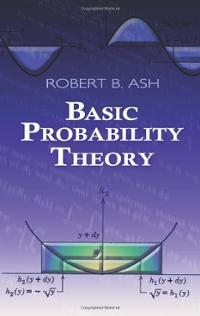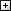Processing ......FreeComputerBooks.com Free Computer, Mathematics, Technical Books and Lecture Notes, etc.

Basic Probability Theory
• Title Basic Probability Theory
• Author(s) Robert B. Ash
• Publisher: Dover Publications (June 26, 2008)
• Hardcover/Paperback 352 pages
• eBook PDF Files, and a single PDF (78 MB)
• Language: English
• ISBN-10/ASIN: 0486466280
• ISBN-13: 978-0486466286Book Description

This introduction to more advanced courses in probability and real analysis emphasizes the probabilistic way of thinking, rather than measure-theoretic concepts.

Geared toward advanced undergraduates and graduate students, its sole prerequisite is calculus, this introductory text surveys random variables, conditional probability and expectation, characteristic functions, infinite sequences of random variables, Markov chains, and an introduction to statistics. Complete solutions to some of the problems appear at the end of the book.

Taking statistics as its major field of application, the text opens with a review of basic concepts, advancing to surveys of random variables, the properties of expectation, conditional probability and expectation, and characteristic functions. Subsequent topics include infinite sequences of random variables, Markov chains, and an introduction to statistics. Complete solutions to some of the problems appear at the end of the book.

Reviews, Ratings, and Recommendations: Related Book Categories: Read and Download Links:Similar Books:All CategoriesRecent BooksIT Research LibraryMiscellaneous BooksComputer LanguagesComputer ScienceData Science/DatabasesElectronic EngineeringJava and Java EE (J2EE)Linux and UnixMathematicsMicrosoft and .NETMobile ComputingNetworking and CommunicationsSoftware EngineeringSpecial TopicsWeb Programming